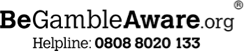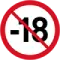New customers only | Commercial content | 18+ age limit | T&Cs apply

# The Kelly Criterion: A winning formula for bettingOne of the most popular strategies bettors adopt to maximise long-term profits is referred to as the Kelly Criterion. This formula, developed by American mathematician John Larry Kelly Jr. in 1956, provides a rational and scientific method for determining the optimal amount to place on a bet. In the following article, we will explore how Kelly's criterion works and how it can be used successfully in the world of betting.

## Kelly Criterion: What is it?

The Kelly Criterion for sports betting is considered a more reliable strategy than others due to it being based on precise mathematical calculations. Compared to methods derived from intuition or luck, the Kelly Criterion offers a rational and calculated approach to managing risk and gaining an advantage in the betting world.

The goal is to use probability theory to determine the ideal bankroll to invest in one's bet and thus intelligently manage one's gaming budget. To perform this calculation, the following four parameters must be defined:

• The total bankroll available
• The match you want to bet on
• The odds of a result coming true
• The odds on which you want to bet

The total bankroll available will determine the amount you are to bet for an individual ticket. Usually, the application of the Kelly Criterion to betting is used by experienced players for proper money management. This is because a careful assessment of the betting event and the odds of a given prediction coming true is required. In addition to experience with the world of betting, it is also important to have a thorough knowledge of the sport one decides to bet on.

## How does the Kelly Criterion work?

The Kelly Criterion for betting is based on a mathematical formula that takes into account the probability of winning and losing, as well as the ratio between winning and losing. The formula is as follows: f* = (bp - q) / b

Where:

• f* represents the fraction of capital to be wagered
• b is the decimal odds of the bet - 1 (eg. odds of 3.25 would become 2.25)
• p is the probability of winning (eg. 65% = 0.65)
• q is the probability of losing (eg. 1 - 0.65 = 0.35)

Kelly's formula calculates the fraction of capital to be bet, so as to maximise the expected return – especially over the long term. Essentially, the criterion suggests betting a percentage of the capital proportional to the advantage one has over the risk. If the result of the formula is positive, it means that there is an advantage in making that bet, otherwise it is preferable to abstain.

The Kelly Criterion could therefore be a good method for understanding when it is worth placing a bet, as well as a strategy for managing one’s gambling budget (ie. bankroll). The success of this method lies in its rationality, provided of course that one's calculations are correct. However, as we shall see, it must be used with caution to avoid losing too much money.

## How to apply the Kelly Criterion?

The Kelly Criterion is a formula that, in order to be used correctly, needs to have defined the probability of winning and losing a bet, as well as the betting odds. Furthermore, it is crucial to be aware of the risks and uncertainties associated with gambling.

Once this data is obtained, the Kelly Criterion can be applied to calculate the fraction of capital to be wagered. For example, if the prediction you want to bet on has decimal odds of 3.00 (b = 2.00) and you determine it has a 40% probability of winning winning (p = 0.40), the calculation would be:

• f* = (2.00 * 0.40 - 0.60) / 2

Which comes out to f* = 0.10 or 10%. In this case, the Kelly Criterion presumes that your edge on this bet is large enough where you can wager 10% of your current available bankroll.

However, it is important to emphasise that one should only proceed with one's bet when f > 0, i.e. when the result obtained is greater than 0. If the result of the calculation is 0 or a negative number, the formula suggests passing on the bet because you will not have favourable odds based on your predicted winning probability. In this case you can either consider betting on the opposite side or simply choose another market or event to bet on.

## Kelly's formula for betting

Kelly's formula can be applied to different types of bets, both in sports and financial betting. However, it is important to note that the Kelly Criterion works best when one has accurate information on the odds and especially the win/loss probabilities. In addition, it is crucial to manage risk carefully and not to bet too high amounts, even if the formula suggests a significant percentage.

### A practical example

Let's take the Inter Milan Champions League semi-final as an example. It is a match in which the Nerazzurri were favourites at 2.10 according to the bookmakers. The odds therefore suggest that Inter have a 47.61% chance of winning against Milan's 27.7% (odds of 3.60).

However, player analysis may show that Inter's true chances are significantly higher, with a 55% chance of beating the Rossoneri. Returning to our example, the player's calculated probability corresponds to a value of around 1.82 in the odds.

Using the Kelly criterion, the calculation to be made would be as follows:

• f* = (1.10 * 0.55 - 0.45) / 1.10

Which comes out to f* = 0.14, or 14%. This means that in this situation, the percentage of capital to bet would be 14% of the established bankroll.

### How to calculate the percentages of a bet

Let's now see how to calculate the probability of an event occurring as a percentage, starting with the odds proposed by the bookmakers, again for the Inter vs AC Milan semi-final. For a 1X2 three-way moneyline market where 1 is the home team, 2 is the away team and X is the draw. An Inter win (1) is offered at 2.10, while the price for the Rossoneri to win (2) is quoted at 3.60. The draw (X) comes in at odds of 3.20.

The calculation to be made is to divide 100 by each odds. The number obtained will be the probability in percentage terms:

• 100 / 2.10 = 47.61 (Home win)
• 100 / 3.20 = 31.25 (Draw)
• 100 / 3.60 = 27.7 (Away win)

## What are the pros and cons of the Kelly Criterion applied to betting?

The Kelly Criterion has several advantages when it comes to betting. First of all, it provides a clear and mathematically based strategy for managing bets efficiently. This can help players make rational decisions and avoid impulsive betting. Furthermore, Kelly's equation has been extensively studied and tested, proving its effectiveness in maximising bankroll management in the long run, provided of course that it is used in the right way.

However, there are also some drawbacks to consider. The Kelly Criterion assumes that the probabilities of winning and losing are precisely known, which is simply not possible in the real world when dealing with sporting events. Furthermore, the formula might suggest bets that require large amounts of one’s bankroll, which might be too much of a risk for some players.

Finally, Kelly's formula does not take into account other important factors, such as the management of one's bankroll and betting volatility. In conclusion, the Kelly Criterion offers a mathematical benchmark for managing bets and maximising long-term profits. However, it is important to use it with caution, taking into account its limitations and one's financial capabilities. As with any form of gambling, risk management and prudence remain key to a positive betting experience.

## When is it appropriate to use the Kelly Criterion on bookmakers?

The Kelly method is a recommended approach to money management for players who have access to accurate information about the odds and the win/loss probability. If you have an advantage over risk by betting on a particular event and have reliable estimates of the probability of winning, then the Kelly Criterion can be applied successfully.

The Kelly Criterion is indeed suitable for players who adopt a rational mindset and make decisions based on mathematical calculations. This approach offers a clear and objective strategy for managing the bankroll for each bet, avoiding impulsive and irrational betting.

However, it is important to carefully consider each variable, including one's goals and risk tolerance, before using this method. If you are risk-averse or prefer to adopt a more conservative strategy, you can use a smaller fraction of Kelly's mathematical formula to reduce your risk exposure.

In conclusion, it makes sense to use the Kelly Criterion when you have accurate information, have a rational mindset and are prepared to accept risk management based on mathematical calculations. It is important to emphasise again that this is not a 100% winning formula, as there is no such thing in the world of betting. Although it is an approach with demonstrable results, it is always important to adapt it to one's own needs and financial capabilities.

## Kelly's Formula: Our tips for sports betting

Here is a series of strategic tips for making the best use of the Kelly Criterion when applying it to sports betting:

• Limit the maximum amount of your bets in order not to risk too large a loss.
• Set a ceiling (e.g. 1 to 5% of your bankroll) or multiply the percentages calculated with the Kelly formula by a coefficient between 0 and 5.
• Set limits: even if personal convictions lead you to bet larger sums, you should limit yourself to the bets calculated with this system. Kelly's formula pays off in the long run!
• Always remember that the sole purpose of the Kelly Criterion is to be able to predict the results of an event more correctly than the bookmakers. With SportyTrader you are in safe hands!

## More about sports betting methods